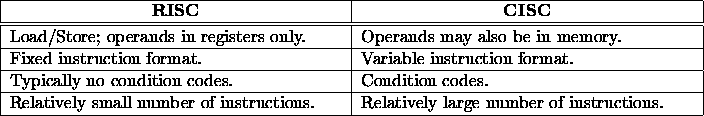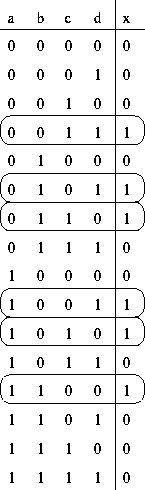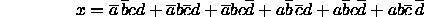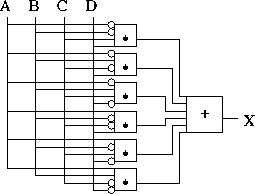# Midterm 1 Solution

CS 26

Oct. 3, 1996)

1. (26 pts.) Compare and contrast the RISC and CISC design styles.2. (36 pts.) Assuming the following:
• r5 contains the value 1000.
• r6 contains the value 1003.
• The label var refers to a data segment memory word in memory location 2000.
• Memory location 1000 contains the value 1.
• Memory location 1001 contains the value 2.
• Memory location 1002 contains the value 3.
• Memory location 1003 contains the value 4.

State the addressing mode used and determine the effective address, for memory references, for each of the following.

1. `#63`

2. `var`

Direct. EA = 2000.

3. `(r5)`

Indirect. EA = 1000.

4. `12(r6)`

Indexed. EA = 1015.

5. `+(r6)`

(Pre)Autoincrement. EA = 1004.

6. If an architecture only offers indexed addressing, offering neither direct nor indirect addressing, it is possible that the assembly language can still offer direct and indirect addressing. Explain how this is so.

Recall that indexed addressing specifies the effective address as the sum of a register and a constant. Indirect addressing is achieved by using a constant value of 0. Direct addressing is achieved by using a register whose value is 0 (note that the range of the constant value might not be the entire memory space).

3. (35 pts.) Interpret, at a high level, this MIPS R2000 assembly language subroutine:
```            .text
subr:       lb \$t0, 0(\$a0)
while:      beqz \$t0, ewhile
bne \$t0, \$a1, next
move \$v0, \$a0
jr \$31
lb \$t0, 0(\$a0)
b while
ewhile:     li \$v0, 0
jr \$31
```
Hints:
• One of the parameters is the address of a 0 terminated string.
• The other parameter is an ASCII character.
• The subroutine returns a pointer.
• The NULL pointer has a value of 0.

It's C's strchr() which searches for a character, c, in a string, s, returning a pointer to the first occurrence of c in s. If c is not be be found in s, the function returns the NULL pointer.

4. (25 pts.) Convert the following fragment of C code to assembly language:
```if (a <= b && a <= c)
min = a;
else if (b <= c)
min = b;
else
min = c;
```
a, b, and c are integers stored in memory and the underlying architecture is load/store.

```            lw \$t0, a(\$0)
lw \$t1, b(\$0)
lw \$t2, c(\$0)
bgt \$t0, \$t1, elseif
bgt \$t0, \$t2, elseif
sw \$t0, min(\$0)
b endif
elseif:     bgt \$t1, \$t2, else
sw \$t1, min(\$0)
b endif
else:       sw \$t2, min(\$0)
endif:
```

5. (20 pts.) Design a circuit which has four inputs and a single output. The output should be true (1) when exactly two of the inputs is true.
1. Write the truth table for the circuit and circle the minterms.2. Write the sum-of-products equation corresponding to the truth table.3. Using combinational logic gates, implement the sum-of-products equation.6. (33 pts.)

1. To what do caller-save and callee-save refer?

This refers to which function is ``responsible'' for saving registers (in an activation record) on a function call --- the caller or callee. For caller-save, the calling function must save any registers which are in-use at the time of the call and restore them after the call. For callee-save, the called function must save any registers which it will use during the call and restore them before returning.

2. What are the two ways for parameters to be passed in assembly language programs?

Through registers and on the stack execution stack.

3. What is an activation record and why is it useful?

An activation record is an organized mechanism for keeping information related to subroutine calls. Such information includes the return address, storage for local variables, storage for saved registers, parameters, and activation linkage information.

The methodology is useful because it provides for recursion.

Thomas P. Kelliher
Tue Oct 8 13:41:07 EDT 1996
Tom Kelliher